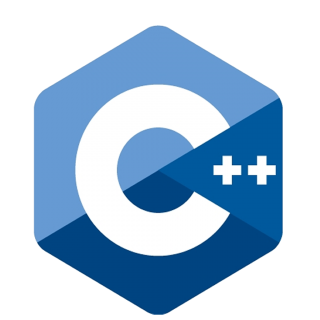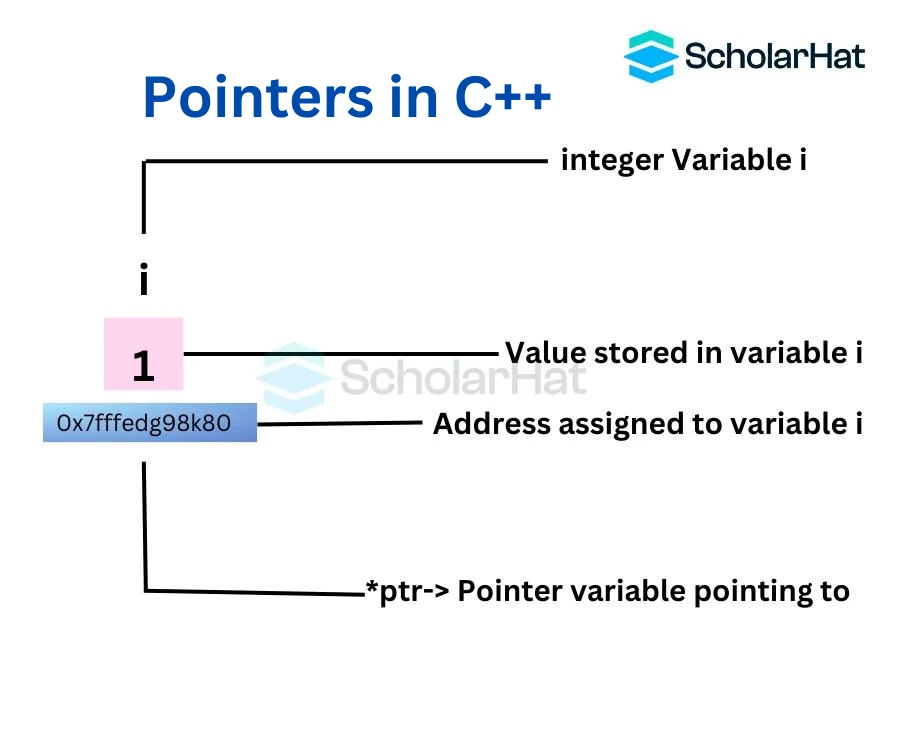# Pointers in C++: Declaration, Initialization and Advantages

25 Nov 2023
Intermediate
1.25K ViewsLearn via Video Course & by Doing Hands-on Labs

## Pointers in C++: An Overview

In this comprehensive C++ tutorial, we'll take you on a guided tour of the C++ language, from its foundational concepts to the advanced ones. In addition to the insights shared in this article, consider taking advantage of C++ Online Training to enhance your knowledge and proficiency. We'll provide the information on pointers that will not only help build your knowledge in C++ language

.

## What are Pointers in C++?

Pointers are `variables` that contain the memory address of another `variable` instead of a direct value. Such variables can be of type `int`, `float`, `char`, etc. By referencing this memory address, programmers can access and modify data indirectly.## Use of pointers in C++

• Dynamic memory allocation - In the C++ programming language, the programmers can dynamically allocate memory by using the `malloc()` and `calloc()` functions particularly where the pointer is used. This is the primary use of pointers in C++
• Arrays, Functions, and Structures - Pointers in the c ++ programming language are widely used in `arrays`, `structures`, and `functions`. It reduces the code and improves the performance of that particular program.

### Pointer Declaration in C++

We do not initialize the pointer; we simply declare it using the `*` (asterisk symbol) before a pointer's name or after the data type.

#### Syntax

• ``datatype *var_name;``
• ``````datatype* var_name; //preferred syntax
``````

#### Example

• ``int *a;//pointer to int ``
• ``int* a;//pointer to int ``

### Pointer Initialization in C++

The procedure of initializing a pointer is where we give the memory address of another variable using the `&` (address of) operator.

#### Example

``````#include <iostream>
using namespace std;

int main() {
int x = 42; // Declare and initialize an integer variable 'x'

// declare pointer variable 'ptr' and initialize it with the address of 'x'
int *ptr = &x;

// print value of x
cout << "x = " << x << endl;

cout << "Address of x (&x) = " << &x << endl
<< endl;

// print pointer ptr
cout << "ptr = " << ptr << endl;

// print the content of the address ptr points to
cout << "Content of the address pointed to by ptr (*ptr) = " << *ptr << endl;

return 0;
}
``````

In this C++ code,

• an `integer` variable, `x` is initialized to 42
• a pointer, `ptr` is created to hold `x's` address in memory.

Output

``````x = 42
Address of x (&x) = 0x7ffeb5f7b3c4

ptr = 0x7ffeb5f7b3c4
Content of the address pointed to by ptr (*ptr) = 42
``````

`*` used in the above example can confuse you. It serves two purposes:

• When used in declaration (`int *a`), it creates a pointer variable.
• When not used in declaration (`*a`), it act as a dereference operator.

### Modifying Values Pointed by Pointers

#### Example

``` ```#include <iostream>
using namespace std;

int main() {
int x = 42; // Declare and initialize an integer variable 'x'

// declare pointer variable 'ptr' and initialize it with the address of 'x'
int *ptr = &x;

// print value of x
cout << "x = " << x << endl;

// print *ptr
cout << "*ptr = " << *tr << endl
<< endl;

cout << "Changing value of x to 70:" << endl;

// change value of x to 70
x = 70;

// print x
cout << "x = " << x << endl;

// print *ptr
cout << "*ptr = " << *ptr << endl
<< endl;

cout << "Changing value of *ptr to 60:" << endl;

// change value of ptr to 60
*ptr = 60;

// print x
cout << "x = " << x << endl;

// print *ptr
cout << "*ptr = " << *ptr << endl;
return 0;
}
``````

In the above C++ code, `ptr` points to the address of `x`. Therefore

• when value of `x` is changed, `*ptr` also gets changed
• when `*ptr` is modified, value of `x` also gets modified

Output

``````x = 42
*ptr = 42

Changing value of x to 70:
x = 70
*ptr = 70

Changing value of *ptr to 60:
x = 60
*ptr = 60``````

Read more: Call by Value and Call by Reference in C++

## Advantages of using Pointers in C ++

There are a few advantages to using `pointers` in C ++
• Pointers help to reduce the code and improve the performance of the program. It mainly assists in retrieving `trees`, `strings`, and many more. Pointers are primarily used with `arrays`, `functions`, and `structures`.
• The programmer or user can return multiple values from a particular function with the help of the `pointer`
• `Pointers` help users access any memory location in the memory of the computer.

## Summary

Pointers are a powerful tool in C++, but they can be difficult to use correctly. If you're new to pointers, start by reading this C++ Certification Training guide and experimenting with them in your programs. Your ability to code for effective and feature-rich programs will improve as a result of understanding C++ `pointers` and practicing with examples.

Share Article
Similar Articles